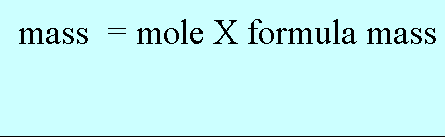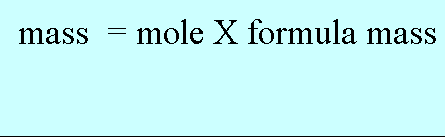Formula mass Before we go into calculations of the formula mass, it is important to point out a very valuable mathematical tool called transposition. It simply means changing the formula around to make it equal to something else. This simple technique prevents us having to memorise many, many complex formulae. We know the formula used to calculate the mass of substance if we know the number of moles and its formula mass. m = n X M Now we can transpose this formula to make the formula mass(M) the subject. We can do this two ways , mathematically or visually. Mathematically. Divide both sides of the equation by the number of moles. This removes the moles from the right hand side to leave only the formula mass.Visually. Remove the moles from the right hand side by taking across the "=" sign and doing the opposite operation. In this case we are multiplying the moles on the right so when we cross the "=" sign it changes to division and goes at the bottom.Home